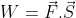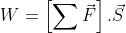# Important Work Power and Energy Formulas For JEE Main and Advanced

On this page, students will find a compilation of all the formulas related to the topic of work, energy and power. The list of formulas will help students to revise all the important notes quickly before the entrance exams.

## Work, Power and Energy Formulas

1. Work Done by Constant ForceW = FS cos Ө

It is a scalar quantity

2. Work done by Multiple ForcesW = W1 +W2+W3+….

Work done on the particle is the sum of the individual work done by all the forces acting on the particles.

3. Dimensions of work

Work = [ML2T-2]

4. Kinetic Energy

As mass is a positive quantity kinetic energy is a positive quantity.

K = ½ mv2= p2/2m

p is the linear momentum

5. Potential Energy

F = -dU/dx for conservative forces

UGravitational = mgh

Uspring =(1/2) kx2

6. Work energy theorem

According to the theorem, the change in kinetic energy of a body is the net work done by the net force on the body

W= ∆K

7. Mechanical energy

Mechanical energy E of an object or a system is the sum of kinetic energy K and potential energy U.

E= U+K. Conserved if forces are conservative in nature

8. Power

Pav = W/t ,

Here W is the work done and t is the time taken

Test Your Knowledge On Important Work Power And Energy Formulas For Jee!